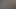Back to Blog

# Project Calculation Formulae

08 Mar 2021 · v1.0.1 · Prof. Dr. Ulrich AndersHere are the most relevant formulae for calculating project investment ratios with the used definitions:

\begin{aligned} \text{PV} &= \sum_{t=0}^T { \text{CF}_t \times \text{DF}_t} \\\\ \text{NPV} &= \sum_{t=0}^T { (\text{RCF}_t + \text{ICF}_t) \times \text{DF}_t} \\\\ \text{FV}_T &= \sum_{t=0}^T { \text{CF}_t \times \text{AF}_t} \\\\ \text{DF}_t &= { 1 \over (1 + \text{i}_t)^t} \\\\ \text{AF}_t &= (1 + \text{i}_t)^{(T-t)} \\\\ \text{NPV}_\text{IRR} \stackrel{!}{=} 0 &= \sum_{t=0}^T { \text{CF}_t \times {1 \over (1 + \text{IRR})^t}} \\\\ \text{Excel: } \text{MIRR} &= \sqrt[T]{\frac{\text{FV}_{T,r} \text{[positive CFs]}}{-\text{PV}_f\text{[negative CFs]}}} - 1 \\\\ \text{better: } \text{MIRR} &= \sqrt[T]{\frac{\text{FV}_{T,r} \text{[RCFs]}}{-\text{PV}_f\text{[ICFs]}}} - 1 \\\\ \text{ROI} &= \frac{\text{PV}\text{[RCFs]}}{-\text{PV}\text{[ICFs]}} -1 \\\\ \end{aligned}
\begin{aligned} \text{CF} &= \text{Cash Flow} \\ \text{RCF} &= \text{Return Cash Flow (positive)} \\ \text{ICF} &= \text{Investment Cash Flow (negative)} \\ \text{PV} &= \text{Present Value} \\ \text{NPV} &= \text{Net Present Value} \\ \text{FV} &= \text{Future Value} \\ \text{DF} &= \text{Discount Factor} \\ \text{AF} &= \text{Accumulation Factor} \\ \text{IRR} &= \text{Internal Rate of Return} \\ \text{MIRR} &= \text{Modified Internal Rate of Return} \\ \text{ROI} &= \text{Return on Investment} \\ i &= \text{interest rate}\\ f &= \text{financing rate}\\ r &= \text{re-investment rate} \\ \text{t} &= \text{time period} \\ \text{T} &= \text{final time period} \\ \end{aligned}

## Be careful with NPV and MIRR in Excel

• Be careful if you use NPV in Excel. Normally, net present value (NPV) means that you are netting the present value (PV) of the return cash flows (CFs) from period 1 and later with the investment in period 0. However, the NPV-function in Excel only calculates the present value of the return cash flows from period 1 and later. You have to net this PV with the investment in period 0 yourself in order to get the NPV.

• Be careful if you use the definition of MIRR from Excel. Excel-MIRR takes the bold assumption, that all cash flows that are negative are investments. But not all cash flows that are negative can be considered investments. So it is better to calculate MIRR yourself. You also should not net negative investments with positive return cash flows from the same period. This also distorts the Excel-MIRR.